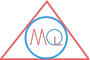# Surface Area and Volume Formulas of 3-D Shapes

## Surface area formulas of 3-D shapes

PolygonSurface Area
Cylinder$$2 \pi r h$$
Cone$$\pi r l$$
Sphere$$4 \pi r^2$$
Hemisphere$$2 \pi r^2$$

## Total surface area formulas of 3-D shapes

PolygonTotal Surface Area
Cuboid$$2(l b + b h + h l)$$
Cube$$6 (l)^2$$
Cylinder$$2 \pi r(r + h)$$
Cone$$\pi r(l + r)$$
SphereNA
Hemisphere$$3 \pi r^2$$

NA $$\implies$$ not applicable

## Volume formulas of 3-D shapes

PolygonVolume
Cuboid $$l \times b \times h$$
Cube$$(l)^3$$
Cylinder$$\pi r^2 h$$
Cone$$\frac{1}{3} \pi r^2 h$$
Sphere$$\frac{4}{3} \pi r^3$$
Hemisphere$$\frac{2}{3} \pi r^3$$